# two indicators in one simple EA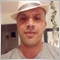41

Hello

I am a great beginner, I am not a developer. But I took up the challenge of creating an EA.

For several days now, I have had a lot of difficulty making progress.

IHello

I am a great beginner, I am not a developer. But I took up the challenge of creating an EA.

For several days now, I have had a lot of difficulty making progress.

I just want to create an EA that is able to work with two indicators at the same time: the RSI and the TriX.

(For example :

if the rsi = 30 and trix = 0.0001 do that ...)

I manage to get the last value of the RSI, but I struggle to get that of the TriX ...

I have read a lot of topics on forums and technical docs, but I can't seem to find the solution

Here is the EA code,

```//+------------------------------------------------------------------+
//|                                                rsi-trix-test.mq5 |
//|                                                     Hamdi FOUZAI |
//|                                             https://www.mql5.com |
//+------------------------------------------------------------------+
#property version   "1.00"

#include <GetIndicatorBuffers.mqh>

/*

the purpose of this code is to get
the current RSI and TRIX to be able
to code conditions and actions
however, I cannot get the last value of the TRIX

*/

//+--------------+
//| RSI Array    |
//+--------------+

//---- arrays for indicators
double      RSI[];                // array for the indicator iRSI
//---- handles for indicators
int         RSI_handle;           // handle of the indicator iRSI

//+--------------+
//| TRIX Array   |
//+--------------+

//---- arrays for indicators
double      TriX[];                // array for the indicator iTriX
//---- handles for indicators
int         TriX_handle;           // handle of the indicator iTriX

//////////////////////////////////////////////////////////////////////
//+------------------------------------------------------------------+
//| Expert initialization function                                   |
//+------------------------------------------------------------------+
//////////////////////////////////////////////////////////////////////

int OnInit()
{
//---

//+--------------+
//| RSI          |
//+--------------+

//--- creation of the indicator iRSI
RSI_handle=iRSI(NULL,0,21,PRICE_CLOSE);
//--- report if there was an error in object creation
if(RSI_handle<0)
{
Print("The creation of iRSI has failed: Runtime error =",GetLastError());
//--- forced program termination
return(-1);
}
return(0);

//+--------------+
//| TRIX         |
//+--------------+

//--- creation of the indicator iTriX
TriX_handle=iTriX(NULL,0,20,PRICE_CLOSE);
//--- report if there was an error in object creation
if(TriX_handle<0)
{
Print("The creation of iTriX has failed: Runtime error =",GetLastError());
//--- forced program termination
return(-1);
}
return(0);

//+------------------+
//| set buffer index |
//+------------------+

SetIndexBuffer(0,RSI,INDICATOR_DATA);
SetIndexBuffer(1,TriX,INDICATOR_DATA);

//---
return(INIT_SUCCEEDED);
}

//////////////////////////////////////////////////////////////////////
//+------------------------------------------------------------------+
//| Expert deinitialization function                                 |
//+------------------------------------------------------------------+
//////////////////////////////////////////////////////////////////////

void OnDeinit(const int reason)
{
//---

}

//////////////////////////////////////////////////////////////////////
//+------------------------------------------------------------------+
//| Expert tick function                                             |
//+------------------------------------------------------------------+
//////////////////////////////////////////////////////////////////////

void OnTick()
{
//---

//+--------------+
//| RSI          |
//+--------------+

//--- filling an array RSI[] with current values of iRSI
//--- set indexation of array RSI[] as timeserie
//--- return if there was an error
if(!CopyBufferAsSeries(RSI_handle,0,0,100,true,RSI)) return;

// I add this line to check that I get the current RSI
// It works
Print("rsi value: ",RSI);

//+--------------+
//| TRIX         |
//+--------------+

//--- filling an array TriX[] with current values of iTriX
//--- set indexation of array TriX[] as timeseries
//--- return if there was an error
if(!CopyBufferAsSeries(TriX_handle,1,0,100,true,TriX)) return;

// I add this line to check that I get the current TRIX
// It doesn't works
Print("trix value: ",TriX);

}
//+------------------------------------------------------------------+
```
Files: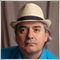5787

Your code does not even compile and you are mixing concepts of EAs and Indicators.

• Don't use the function "SetIndexBuffer()" in EAs. They are for Indicators.
• The function "CopyBufferAsSeries()" does not exist in MQL5. You probably meant to use "CopyBuffer()".
• However, for "CopyBuffer()", the parameters you are using don't match for that function. There is an extra boolean parameter.5787

You probability wanted something like this maybe?

```if(!CopyBuffer(RSI_handle,0,0,100,RSI)) return;
Print("rsi value: ",RSI);

if(!CopyBuffer(TriX_handle,1,100,100,TriX)) return;
Print("rsi value: ",TriX);```5787

By the way, the function "CopyBufferAsSeries" is a user function (not a built-in function), created and described in following Article: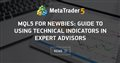MQL5 for Newbies: Guide to Using Technical Indicators in Expert Advisors
• www.mql5.com
In order to obtain values of a built-in or custom indicator in an Expert Advisor, first its handle should be created using the corresponding function. Examples in the article show how to use this or that technical indicator while creating your own programs. The article describes indicators that are built n the MQL5 language. It is intended for those who don't have much experience in the development of trading strategies and offers simple and clear ways of working with indicators using the offered library of functions.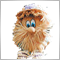Moderator
251596

### An example of get values from the iRSI indicator

Code: iRSI get value.mq5

Do not forget the rules: create the indicator handle ONCE in OnInit,

```//+------------------------------------------------------------------+
//| Expert initialization function                                   |
//+------------------------------------------------------------------+
int OnInit()
{
//--- create handle of the indicator iRSI
handle_iRSI=iRSI(Symbol(),Period(),Inp_RSI_ma_period,Inp_RSI_applied_price);
//--- if the handle is not created
if(handle_iRSI==INVALID_HANDLE)
{
//--- tell about the failure and output the error code
PrintFormat("Failed to create handle of the iRSI indicator for the symbol %s/%s, error code %d",
Symbol(),
EnumToString(Period()),
GetLastError());
//--- the indicator is stopped early
return(INIT_FAILED);
}
//---
return(INIT_SUCCEEDED);
}```

use CopyBuffer to get the data:

```//+------------------------------------------------------------------+
//| Expert tick function                                             |
//+------------------------------------------------------------------+
void OnTick()
{
//---
double rsi[];
ArraySetAsSeries(rsi,true);
int start_pos=0,count=2;
if(!iGetArray(handle_iRSI,0,start_pos,count,rsi))
return;
//---
string text="";
int limit=(count>2)?2:count;
for(int i=0; i<limit; i++)
{
text=text+
" bar #"+IntegerToString(i)+": "+
" rsi "+DoubleToString(rsi[i],2)+"\n";
}
Comment(text);
}```

Result: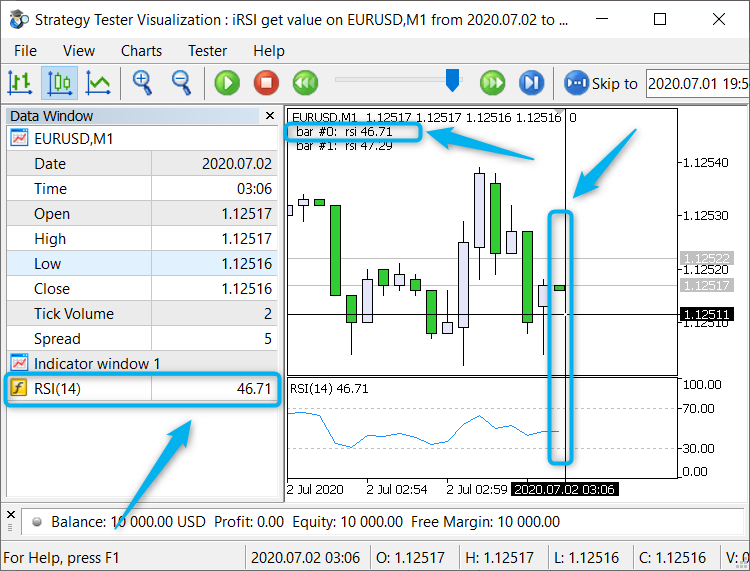Рис. 1. iRSI get value41

Fernando Carreiro:

Your code does not even compile and you are mixing concepts of EAs and Indicators.

• Don't use the function "SetIndexBuffer()" in EAs. They are for Indicators.
• The function "CopyBufferAsSeries()" does not exist in MQL5. You probably meant to use "CopyBuffer()".
• However, for "CopyBuffer()", the parameters you are using don't match for that function. There is an extra boolean parameter.

Thank you very much for your support

My code compile with no errors in my editor, I use "MetaEditor Version 5.00 build 2885 14 April 2021"

here is the result of the code in the strategy tester

I get the RSI value in the console but not the TRIX (see the picture capture-1.jpg attached file)

I modified the code in the OnTick() section with CopyBuffer, as you explain :

```//////////////////////////////////////////////////////////////////////
//+------------------------------------------------------------------+
//| Expert tick function                                             |
//+------------------------------------------------------------------+
//////////////////////////////////////////////////////////////////////

void OnTick()
{
//---

//+--------------+
//| RSI          |
//+--------------+

if(!CopyBuffer(RSI_handle,0,0,100,RSI)) return;
Print("rsi value: ",RSI);

//+--------------+
//| TRIX         |
//+--------------+

if(!CopyBuffer(TriX_handle,1,100,100,TriX)) return;
Print("Trix value: ",TriX);
}```

But now when I test it,

• TriX value is always 0
• Rsi value is not the sama as the graph

(see capture-2.jpg attached)

maybe do you know where I am wrong? thank you for your help

Files:
Capture-1.jpg  431 kb
Capture-2.jpg  412 kb5787

Hamdi Fouzai: Thank you very much for your support. My code compile with no errors in my editor, I use "MetaEditor Version 5.00 build 2885 14 April 2021"

here is the result of the code in the strategy tester  I get the RSI value in the console but not the TRIX

We are unable to compile your code because you have not supplied all the necessary files. Your code depends on an include files which you did not supply, namely "GetIndicatorBuffers.mqh".

Since we did not have that file, all we can do is guess and give you advice based on what was given.

First correct the errors described by me (about "SetIndexBuffer" ) and also by Vladimir Karputov about the Indicator handles being initiallised properly in OnInit and then try again, to see what changes.

Should it not be resolved, then supply all files with your new changes.41

Fernando Carreiro:

We are unable to compile your code because you have not supplied all the necessary files. Your code depends on an include files which you did not supply, namely "GetIndicatorBuffers.mqh".

Since we did not have that file, all we can do is guess and give you advice based on what was given.

First correct the errors described by me (about "SetIndexBuffer" ) and also by Vladimir Karputov about the Indicator handles being initiallised properly in OnInit and then try again, to see what changes.

Should it not be resolved, then supply all files with your new changes.

thank you Fernando

here is the getindicatorbuffers.mqh

```//+------------------------------------------------------------------------------+
//|                                                      GetIndicatorBuffers.mqh |
//|                                                          http://www.mql5.com |
//+------------------------------------------------------------------------------+
//+------------------------------------------------------------------------------+
//| Copies values of the indicator to the buffer                                 |
//+------------------------------------------------------------------------------+
bool CopyBufferAsSeries(
int handle,      // handle
int bufer,       // buffer number
int start,       // start from
int number,      // number of elements to copy
bool asSeries,   // is as series
double &M[]      // target array for data
)
{
//--- filling M with current values of the indicator
if(CopyBuffer(handle,bufer,start,number,M)<=0) return(false);
//--- setting ordering for M
//--- åñëè asSeries=true, the indexation of M as timeseries
//--- åñëè asSeries=false, the indexation of M as default
ArraySetAsSeries(M,asSeries);
//---
return(true);
}
//+------------------------------------------------------------------------------+
//| Copies the values of the indicator ADX to the arrays                         |
//+------------------------------------------------------------------------------+
int start,
int number,
double &Main[],
double &PlusDI[],
double &MinusDI[],
bool asSeries=true  // as series
)
{
//--- Filling the array Main with the current values of MAIN_LINE
//--- Filling the array PlusDI with the current values of PLUSDI_LINE
//--- Filling the array MinusDI with the current values of MINUSDI_LINE
//---
return(true);
}
//+------------------------------------------------------------------------------+
//| Copies the values of the indicator ADXWilder to the arrays                   |
//+------------------------------------------------------------------------------+
int start,
int number,
double &Main[],
double &PlusDI[],
double &MinusDI[],
bool asSeries=true  // as series
)
{
//--- Filling the array Main with the current values of MAIN_LINE
//--- Filling the array PlusDI with the current values of PLUSDI_LINE
//--- Filling the array MinusDI with the current values of MINUSDI_LINE
//---
return(true);
}
//+------------------------------------------------------------------------------+
//| Copies the values of the indicator Alligator to the arrays                   |
//+------------------------------------------------------------------------------+
bool GetAlligatorBuffers(int Alligator_handle,
int start,
int number,
double &Jaws[],
double &Teeth[],
double &Lips[],
bool asSeries=true  // as series
)
{
//--- Filling the array Jaws with the current values of GATORJAW_LINE
if(!CopyBufferAsSeries(Alligator_handle,0,start,number,asSeries,Jaws)) return(false);
//--- Filling the array Teeth with the current values of GATORTEETH_LINE
if(!CopyBufferAsSeries(Alligator_handle,1,start,number,asSeries,Teeth)) return(false);
//--- Filling the array Lips with the current values of GATORLIPS_LINE
if(!CopyBufferAsSeries(Alligator_handle,2,start,number,asSeries,Lips)) return(false);
//---
return(true);
}
//+------------------------------------------------------------------------------+
//| Copies the values of the indicator Bands to the arrays                       |
//+------------------------------------------------------------------------------+
bool GetBandsBuffers(int Bands_handle,
int start,
int number,
double &Base[],
double &Upper[],
double &Lower[],
bool asSeries=true  // as series
)
{
//--- Filling the array Base with the current values of BASE_LINE
if(!CopyBufferAsSeries(Bands_handle,0,start,number,asSeries,Base)) return(false);
//--- Filling the array Upper with the current values of UPPER_BAND
if(!CopyBufferAsSeries(Bands_handle,1,start,number,asSeries,Upper)) return(false);
//--- Filling the array Lower with the current values of LOWER_BAND
if(!CopyBufferAsSeries(Bands_handle,2,start,number,asSeries,Lower)) return(false);
//---
return(true);
}
//+------------------------------------------------------------------------------+
//| Copies the values of the indicator Envelopes to the arrays                   |
//+------------------------------------------------------------------------------+
bool GetEnvelopesBuffers(int Envelopes_handle,
int start,
int number,
double &Upper[],
double &Lower[],
bool asSeries=true       // as series
)
{
//--- Filling the array Upper with the current values of UPPER_LINE
if(!CopyBufferAsSeries(Envelopes_handle,0,start,number,asSeries,Upper)) return(false);
//--- Filling the array Lower with the current values of LOWER_LINE
if(!CopyBufferAsSeries(Envelopes_handle,1,start,number,asSeries,Lower)) return(false);
//---
return(true);
}
//+------------------------------------------------------------------------------+
//| Copies the values of the indicator Fractals to the arrays                   |
//+------------------------------------------------------------------------------+
bool GetFractalsBuffers(int Fractals_handle,
int start,
int number,
double &Upper[],
double &Lower[],
bool asSeries=true       // as series
)
{
//--- Filling the array Upper with the current values of UPPER_LINE
if(!CopyBufferAsSeries(Fractals_handle,0,start,number,asSeries,Upper)) return(false);
//--- Filling the array Lower with the current values of LOWER_LINE
if(!CopyBufferAsSeries(Fractals_handle,1,start,number,asSeries,Lower)) return(false);
//---
return(true);
}
//+------------------------------------------------------------------------------+
//| Copies the values of the indicator Gator to the arrays                   |
//+------------------------------------------------------------------------------+
bool GetGatorBuffers(int Gator_handle,
int start,
int number,
double &Upper[],
double &Lower[],
bool asSeries=true       // as series
)
{
//--- Filling the array Upper with the current values of UPPER_LINE
if(!CopyBufferAsSeries(Gator_handle,0,start,number,asSeries,Upper)) return(false);
//--- Filling the array Lower with the current values of LOWER_LINE
if(!CopyBufferAsSeries(Gator_handle,1,start,number,asSeries,Lower)) return(false);
//---
return(true);
}
//+------------------------------------------------------------------------------+
//| Copies the values of the indicator Ichimoku to the arrays                    |
//+------------------------------------------------------------------------------+
bool GetIchimokuBuffers(int Ichimoku_handle,
int start,
int number,
double &Tenkansen[],
double &Kijunsen[],
double &SenkouspanA[],
double &SenkouspanB[],
double &Chinkouspan[],
bool asSeries=true       // as series
)
{
//--- Filling the array Tenkansen with the current values of TENKANSEN_LINE
if(!CopyBufferAsSeries(Ichimoku_handle,0,start,number,asSeries,Tenkansen)) return(false);
//--- Filling the array Kijunsen with the current values of KIJUNSEN_LINE
if(!CopyBufferAsSeries(Ichimoku_handle,1,start,number,asSeries,Kijunsen)) return(false);
//--- Filling the array SenkouspanA with the current values of SENKOUSPANA_LINE
if(!CopyBufferAsSeries(Ichimoku_handle,2,start,number,asSeries,SenkouspanA)) return(false);
//--- Filling the array SenkouspanB with the current values of SENKOUSPANB_LINE
if(!CopyBufferAsSeries(Ichimoku_handle,3,start,number,asSeries,SenkouspanB)) return(false);
//--- Filling the array Chinkouspan with the current values of CHINKOUSPAN_LINE
if(!CopyBufferAsSeries(Ichimoku_handle,4,start,number,asSeries,Chinkouspan)) return(false);
//---
return(true);
}
//+------------------------------------------------------------------------------+
//| Copies the values of the indicator MACD to the arrays                        |
//+------------------------------------------------------------------------------+
bool GetMACDBuffers(int MACD_handle,
int start,
int number,
double &Main[],
double &Signal[],
bool asSeries=true       // as series
)
{
//--- Filling the array Main with the current values of MAIN_LINE
if(!CopyBufferAsSeries(MACD_handle,0,start,number,asSeries,Main)) return(false);
//--- Filling the array Signal with the current values of SIGNAL_LINE
if(!CopyBufferAsSeries(MACD_handle,1,start,number,asSeries,Signal)) return(false);
//---
return(true);
}
//+------------------------------------------------------------------------------+
//| Copies the values of the indicator RVI to the arrays                         |
//+------------------------------------------------------------------------------+
bool GetRVIBuffers(int RVI_handle,
int start,
int number,
double &Main[],
double &Signal[],
bool asSeries=true       // as series
)
{
//--- Filling the array Main with the current values of MAIN_LINE
if(!CopyBufferAsSeries(RVI_handle,0,start,number,asSeries,Main)) return(false);
//--- Filling the array Signal with the current values of SIGNAL_LINE
if(!CopyBufferAsSeries(RVI_handle,1,start,number,asSeries,Signal)) return(false);
//---
return(true);
}
//+------------------------------------------------------------------------------+
//| Copies the values of the indicator Stochastic to the arrays                  |
//+------------------------------------------------------------------------------+
bool GetStochasticBuffers(int Stochastic_handle,
int start,
int number,
double &Main[],
double &Signal[],
bool asSeries=true       // as series
)
{
//--- Filling the array Main with the current values of MAIN_LINE
if(!CopyBufferAsSeries(Stochastic_handle,0,start,number,asSeries,Main)) return(false);
//--- Filling the array Signal with the current values of SIGNAL_LINE
if(!CopyBufferAsSeries(Stochastic_handle,1,start,number,asSeries,Signal)) return(false);
//---
return(true);
}
//+------------------------------------------------------------------------------+```
Files:41

Fernando Carreiro:

First correct the errors described by me (about "SetIndexBuffer" ) and also by Vladimir Karputov about the Indicator handles being initiallised properly in OnInit and then try again, to see what changes.

I don't know where is my errors in the OnInit section, I wrote this code after the tutorial of Vladimir Karputov (in this tutorial there is an example with all indicators, I copy/paste the example of RSI and Trix)5787

Hamdi Fouzai: But now when I test it,

• TriX value is always 0
• Rsi value is not the sama as the graph

(see capture-2.jpg attached) maybe do you know where I am wrong? thank you for your help

Just noticed now, that you are trying to read the second buffer but Trix only has one buffer.

`if(!CopyBuffer(TriX_handle,0,100,100,TriX)) return; // Use "0" not "1"`

You also seem to be using a different start position than the RSI. Its not an error but it does not make much sense. Also make sure you really have that much data available in terms of Bars available.41

With my first code I get only the RSI in the console of the strategy tester (see capture-1.jpg attached file)

With the CopyBuffer modifications, I get a false value of RSI and always no TriX (see capture-2.jpg ) and the code below

```//+------------------------------------------------------------------+
//|                                                rsi-trix-test.mq5 |
//|                                                     Hamdi FOUZAI |
//|                                             https://www.mql5.com |
//+------------------------------------------------------------------+
#property version   "1.00"

#include <Getindicatorbuffers.mqh>

/*

the purpose of this code is to get
the current RSI and TRIX to be able
to code conditions and actions
however, I cannot get the last value of the TRIX

*/

//+--------------+
//| RSI Array    |
//+--------------+

//---- arrays for indicators
double      RSI[];                // array for the indicator iRSI
//---- handles for indicators
int         RSI_handle;           // handle of the indicator iRSI

//+--------------+
//| TRIX Array   |
//+--------------+

//---- arrays for indicators
double      TriX[];                // array for the indicator iTriX
//---- handles for indicators
int         TriX_handle;           // handle of the indicator iTriX

//////////////////////////////////////////////////////////////////////
//+------------------------------------------------------------------+
//| Expert initialization function                                   |
//+------------------------------------------------------------------+
//////////////////////////////////////////////////////////////////////

int OnInit()
{
//---

//+--------------+
//| RSI          |
//+--------------+

//--- creation of the indicator iRSI
RSI_handle=iRSI(NULL,0,21,PRICE_CLOSE);
//--- report if there was an error in object creation
if(RSI_handle<0)
{
Print("The creation of iRSI has failed: Runtime error =",GetLastError());
//--- forced program termination
return(-1);
}
return(0);

//+--------------+
//| TRIX         |
//+--------------+

//--- creation of the indicator iTriX
TriX_handle=iTriX(NULL,0,20,PRICE_CLOSE);
//--- report if there was an error in object creation
if(TriX_handle<0)
{
Print("The creation of iTriX has failed: Runtime error =",GetLastError());
//--- forced program termination
return(-1);
}
return(0);

//+------------------+
//| set buffer index |
//+------------------+

//SetIndexBuffer(0,RSI,INDICATOR_DATA);
//SetIndexBuffer(1,TriX,INDICATOR_DATA);

//---
return(INIT_SUCCEEDED);
}

//////////////////////////////////////////////////////////////////////
//+------------------------------------------------------------------+
//| Expert deinitialization function                                 |
//+------------------------------------------------------------------+
//////////////////////////////////////////////////////////////////////

void OnDeinit(const int reason)
{
//---

}

//////////////////////////////////////////////////////////////////////
//+------------------------------------------------------------------+
//| Expert tick function                                             |
//+------------------------------------------------------------------+
//////////////////////////////////////////////////////////////////////

void OnTick()
{
//---

//+--------------+
//| RSI          |
//+--------------+

if(!CopyBuffer(RSI_handle,0,0,100,RSI)) return;
Print("rsi value: ",RSI);

//+--------------+
//| TRIX         |
//+--------------+

if(!CopyBuffer(TriX_handle,1,100,100,TriX)) return;
Print("Trix value: ",TriX);
}
//+------------------------------------------------------------------+```
Files:
Capture-1.jpg  431 kb
Capture-2.jpg  412 kb